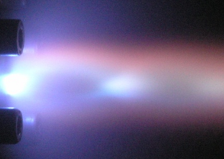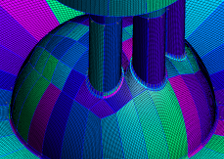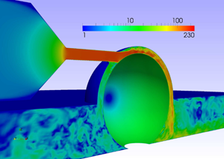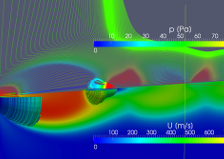### Thermo- and Fluid Dynamics

The lecture ''Thermo- and fluid dynamics'' deals with thermal driven flows and the corresponding changes of density, pressure and temperature. Basic correlations in the fields of gas dynamics and thermo-fluidic behavior of compressible flows are presented. Transonic flows and supersonic shocks are discussed with a causal definition of entropy functions and enthalpy balances. more...### Computational Fluid Dynamics

Computational Fluid Dynamics deals with the prediction of flows through numerical approaches and computational methods. The behavior of technical flows is therefore described through mathematical models. The mathematical problem is written as a system of coupled differential equations. The technical problem is ultimately solved through the correct interpretation of the mathematical solution. more...### Modeling Turbulent Flow

''Modeling turbulent flow'' aims to predict the turbulent flow characteristics in turbulent shear layers and unstable flows. Most of the used models are based on statistical methods for the description of mean velocity profiles and effective momentum diffusion in turbulent flow. The present approaches are characterized by the approximation of turbulent scales and the numerical grid resolution. more...### Micro und Magneto-Fluid Dynamics

''Micro- and Magneto-Fluid Dynamics'' describes a field of study, which deals with molecular motion, inter-molecular forces and the corresponding macroscopic material laws of fluid flow. The ionization process and interaction of charged particles leads to the definition of plasma balance equations. The resulting forces acting on moving charge carriers are described by Magneto-Hydrodynamics (MHD). more...### Relativistic Fluid Dynamics

The lecture "Relativistic fluid dynamics" is a comparison between relativistic and non-relativistic approaches of fluidic phenomena in astrophysics. The used models are derived and discussed for describing astrophysical phenomena like relativistic effects or turbulent diffusion in accretion discs around black holes. more...